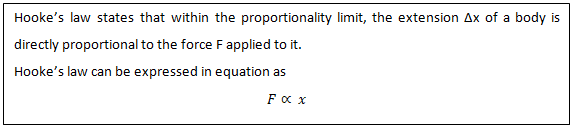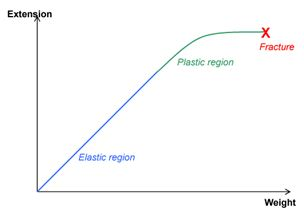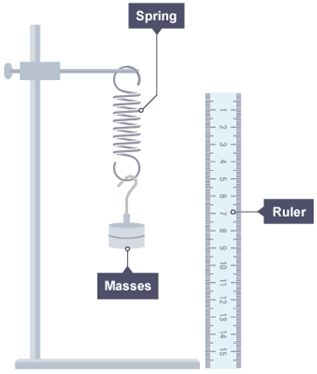# Hooke’s Law

## Introduction

There is one common property behind stretching of a spring and rubber band that come back to their original shape. The property that allows any material to regain its shape is called elasticity. The fundamental law that governs this interesting phenomenon is the Hooke’s law.  In this article, Hooke’s law, a demonstration to understand the law, elastic limit and applications of Hooke’s law are discussed.

Hooke’s Law

Hooke’s law was developed by Robert Hooke in 1676 to describe the direct relationship between force exerted on a spring and its extension. Hooke’s law states that the force (F) exerted on the spring is proportional to its extension (x).A constant of proportionality (k) is inserted in the equation to remove the constant of proportionality,

F = kx

This constant of proportionality is known as spring constant (or force constant).

The spring constant is the force per unit extension. Hence, its unit is (N/m). The spring constant is different for different materials: the larger the value of k, the stiffer the material.

Demonstration of Hooke’s Law

A helical spring, attached to a fixed point, hangs vertically and has weight attached to its lower end as shown in Figure 1. As the magnitude of weight is increased, the spring stretches more and becomes longer. The increase in the length is called the extension and the force (weight) exerted on the spring is called the load.Figure 1: Elasticity

Elastic Limit

For small loads, the spring returns to its original shape once the load is removed. Once the load is increased above a certain limit, the spring fails to return to its original shape and size even after the load is removed. This is the elastic limit of the spring. Beyond the elastic limit, the change in shape is said to be plastic deformation. Some materials have very low elastic limit and they do not obey Hooke’s law at all. Plasticine is an example of such a material. Beyond the plastic region, some brittle materials (e.g. glass, cast iron, etc.) tend to develop cracks and then eventually break. This point is shown in Figure 2 as Fracture.Figure 2: Elastic and Plastic Region

The variation of length of the spring with respect to load is given in Figure 2. The section from origin to the point P is a straight line. In this region, the extension of the spring is directly proportional to the load. The point P is referred to as the proportionality limit. The point E is referred to as the elastic limit as it is usually beyond point P. Beyond point E, the spring deforms permanently and is said to be plastic.The straight line relationship is expressed in Hooke’s law.  Although a spring is described in this case, Hooke’s law holds good to any object, provided the proportionality limit has not been exceeded.

Investigating Hooke’s Law

Let us now investigate Hooke’s law. This will help us understand Hooke’s law scientifically. The apparatus is shown in Figure 3. The aim of this experiment is to find the effect of adding masses on the extension of the spring.Figure 3: Apparatus to Investigate Hooke’s Law

This apparatus is set up. 10 g mass is added to the holder and the extension is recorded. Another 10 g mass is added and the extension is recorded. This procedure is repeated until mass is 50 g. The results are shown in the table below.The spring extended 2 mm each time a 10 g mass is added (which increased the force due to gravity by 0.1 N). This follows Hooke’s law, which states that the extension of an elastic object (like a spring) is directly proportional to the force added.

Plotting the above data in a graph:Finding the gradient of the line using the points (0.2, 4) and (0.4, 8):0.05 N/mm is the spring constant of the spring used in this investigation.

Applications of Hooke’s Law

There are several practical applications to this fundamental law that established a relationship between the load applied to a material and the resultant deformation. Some of the applications are:

1. Design of springs in vehicles
2. Design of weightlifting equipment (cranes and forklifts)

Summary

Hooke’s law deals with a basic concept in physics – elasticity. The widespread applications in various fields makes it an important material behaviour.

• Hooke’s law states that within the proportionality limit, the extension (x) of a body is directly proportional to the force (F) applied to it.
• The elastic limit is the maximum force that can be applied to a wire/spring such that the wire/spring returns to its original length when the load is removed.